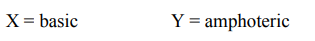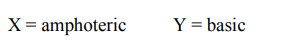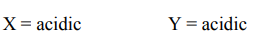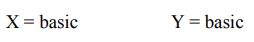# The nature of oxides`
Question:

The nature of oxides $\mathrm{V}_{2} \mathrm{O}_{3}$ and $\mathrm{CrO}$ is indexed as 'X' and 'Y' type respectively. The correct set of X and $\mathrm{Y}$ is:

1.2.3.4.Correct Option: , 4

Solution:

$\mathrm{V}_{2} \mathrm{O}_{3}$ basic

$\mathrm{CrO}$ basic